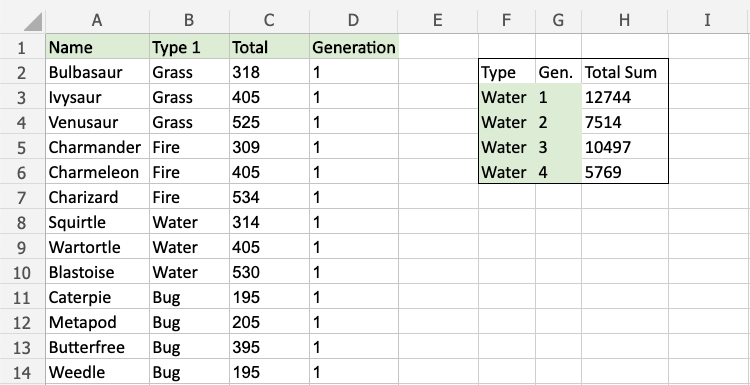# Excel SUMIFS Function

## SUMIFS Function

The SUMIFS function is a premade function in Excel, which calculates the sum of a range based on one or more true or false condition.

It is typed `=SUMIFS`:

=SUMIFS(sum_range, criteria_range1, criteria1, [criteria_range2, criteria2] ...)

The conditions are referred to as `criteria1`, `criteria2`, and so on, which can check things like:

• If a number is greater than another number `>`
• If a number is smaller than another number `<`
• If a number or text is equal to something `=`

The `criteria_range1`, `criteria_range2`, and so on, are the ranges where the function check for the conditions.

The `[sum_range]` is the range where the function calculates the sum.

Note: The different parts of the function are separated by a symbol, like comma `,` or semicolon `;`

The symbol depends on your Language Settings.

## Example SUMIFS function

Find the sum of total stats for Water type 1st Generation Pokemon:

The conditions are that the type is "Water" and Generation is 1.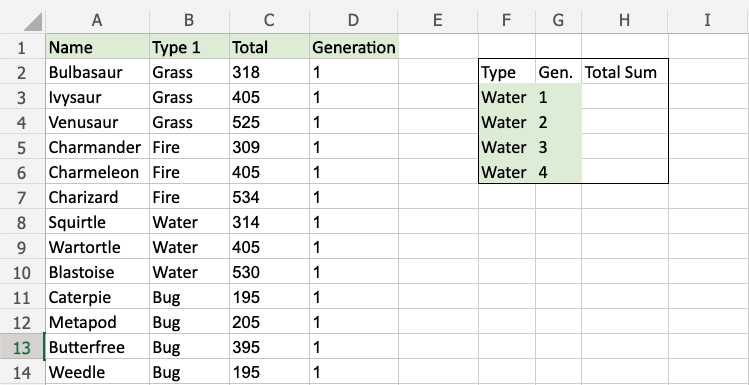Note: The full dataset continues after row 14, all the way down to row 759.

Example SUMIFS function, step by step:

1. Select the cell `H3`
2. Type `=SUMIFS`
3. Double click the SUMIFS command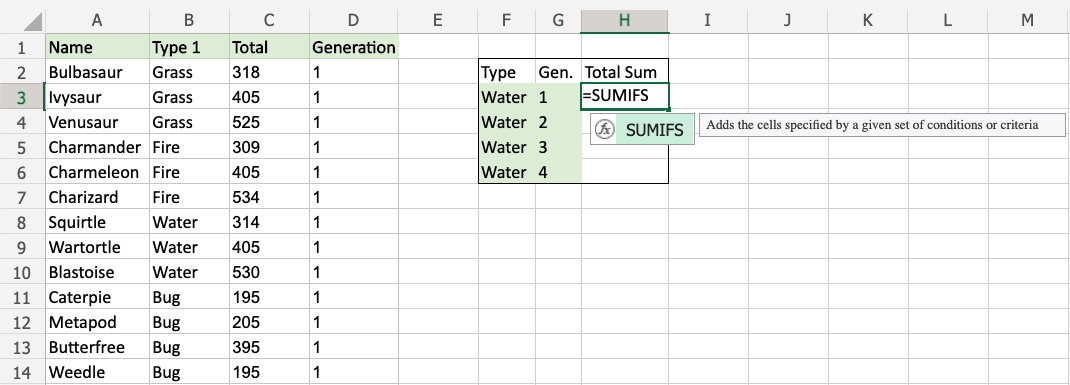1. Specify the range for the sum `C2:C759` (the Total values)
2. Type `,`
3. Specify the range for the first condition`B2:B759` (the Type 1 values)
4. Type `,`
5. Specify the criteria (the cell `F3`, which has the value "Water")
6. Type `,`
7. Specify the range for the second condition`D2:D759` (the Generation values)
8. Type `,`
9. Specify the criteria (the cell `G3`, which has the value "1")
10. Hit enter

Note: You can add more conditions by repeating steps 9-12 before hitting enter.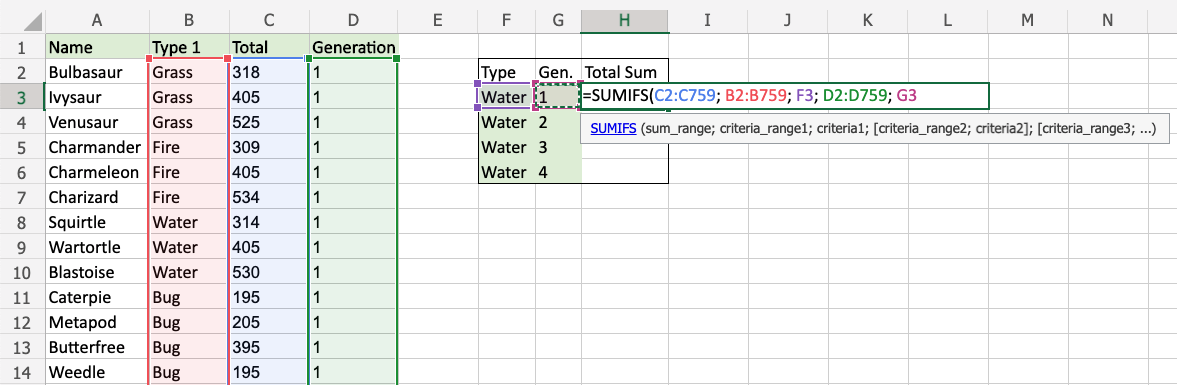The function now calculates the sum of total stats for the 1st Generation Water type Pokemon.

The function can be repeated for the following Generations to compare them: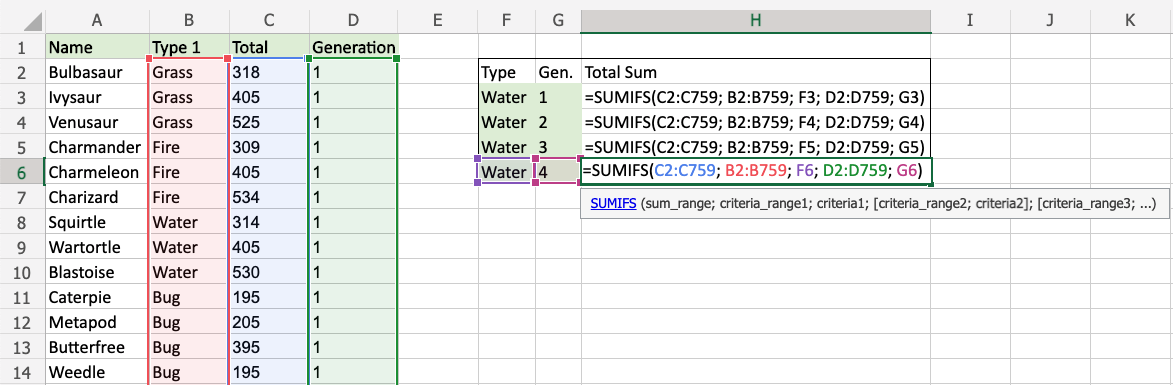Note: You can use the filling function for the other rows, but make sure to use absolute references for the ranges.

Now, we can see the sum of total stats of Water type Pokemon between generations: